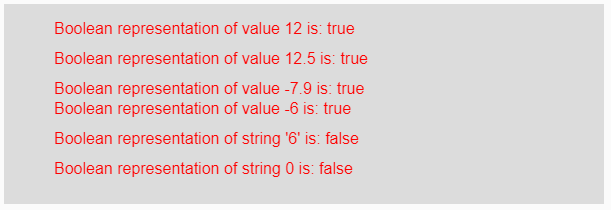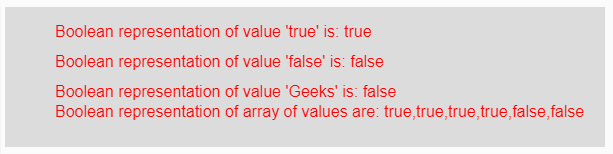# p5.js | boolean() function

The boolean() function in p5.js is used to convert the given string and number value into its boolean representation.

Syntax:

`boolean(Value)`

Parameters: This function accepts single parameter Value which are to be converted into its boolean representation. This value might be integer, float, string, boolean, negative or positive value and array of values.

Return Value: It returns the converted boolean representation.

Below program illustrates the boolean() function in p5.js:

Example 1: This example uses boolean() function to convert input value into its boolean value.

 `function` `setup() {  ` `  `  `    ``// Creating Canvas size ` `    ``createCanvas(600, 200);  ` `}  ` `  `  `function` `draw() {  ` `      `  `    ``// Set the background color  ` `    ``background(220);  ` `    `  `    ``// Initializing some values ` `    ``let Value1 = 12; ` `    ``let Value2 = 12.5; ` `    ``let Value3 = -7.9; ` `    ``let Value4 = -6;  ` `    ``let Value5 = ``"6"``; ` `    ``let Value6 = 0; ` `    `  `    ``// Calling to boolean() function. ` `    ``let A = boolean(Value1); ` `    ``let B = boolean(Value2); ` `    ``let C = boolean(Value3); ` `    ``let D = boolean(Value4); ` `    ``let E = boolean(Value5); ` `    ``let F = boolean(Value6); ` `      `  `    ``// Set the size of text  ` `    ``textSize(16);  ` `      `  `    ``// Set the text color  ` `    ``fill(color(``'red'``));  ` `    `  `    ``// Getting boolean representation ` `    ``text(``"Boolean representation of value 12 is: "` `+ A, 50, 30); ` `    ``text(``"Boolean representation of value 12.5 is: "` `+ B, 50, 60); ` `    ``text(``"Boolean representation of value -7.9 is: "` `+ C, 50, 90); ` `    ``text(``"Boolean representation of value -6 is: "` `+ D, 50, 110); ` `    ``text(``"Boolean representation of string '6' is: "` `+ E, 50, 140); ` `    ``text(``"Boolean representation of string 0 is: "` `+ F, 50, 170); ` `}  `

Output:Example 2: This example uses boolean() function to convert input value into its boolean value.

 `function` `setup() {  ` `  `  `    ``// Creating Canvas size ` `    ``createCanvas(600, 140);  ` `}  ` `  `  `function` `draw() {  ` `      `  `    ``// Set the background color  ` `    ``background(220);  ` `    `  `    ``// Initializing some values ` `    ``let Value1 = ``true``; ` `    ``let Value2 = ``false``; ` `    ``let Value3 = ``"Geeks"``; ` `    ``let Value4 = [12, 3.6, -9.8, ``"true"``, ``false``, ``"Geeks"``]; ` `    `  `    ``// Calling to boolean() function. ` `    ``let A = boolean(Value1); ` `    ``let B = boolean(Value2); ` `    ``let C = boolean(Value3); ` `    ``let D = boolean(Value4); ` `      `  `    ``// Set the size of text  ` `    ``textSize(16);  ` `      `  `    ``// Set the text color  ` `    ``fill(color(``'red'``));  ` `    `  `    ``// Getting boolean representation ` `    ``text(``"Boolean representation of value 'true' is: "` `+ A, 50, 30); ` `    ``text(``"Boolean representation of value 'false' is: "` `+ B, 50, 60); ` `    ``text(``"Boolean representation of value 'Geeks' is: "` `+ C, 50, 90); ` `    ``text(``"Boolean representation of array of values are: "` `+ D, 50, 110); ` `}    `

Output:Note: From the above examples, If the parameter is non-zero value then it returns true and for strings, only “true” string returns true otherwise it returns false output for any other string.

Reference: https://p5js.org/reference/#/p5/boolean

My Personal Notes arrow_drop_upCheck out this Author's contributed articles.

If you like GeeksforGeeks and would like to contribute, you can also write an article using contribute.geeksforgeeks.org or mail your article to contribute@geeksforgeeks.org. See your article appearing on the GeeksforGeeks main page and help other Geeks.

Please Improve this article if you find anything incorrect by clicking on the "Improve Article" button below.

Article Tags :

Be the First to upvote.

Please write to us at contribute@geeksforgeeks.org to report any issue with the above content.